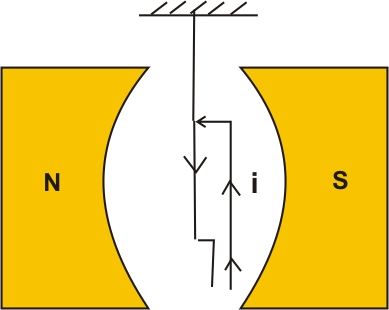# (a) State the underlying principle of a moving coil galvanometer.(b) Give two reasons to explain why a galvanometer cannot as such be used to measure the value of the current in a given circuit.(c) Define the terms : (i) voltage sensitivity and (ii) current sensitivity of a galvanometer.

(a) The underlying principle for the working of a moving coil galvanometer is that when a current-carrying conductor is placed inside a magnetic field, it experiences a magnetic force.When the coil is suspended in a uniform magnetic field it experiences a torque.

Due to this coil rotates and hence, the deflection in the coil of the moving coil galvanometer is directly proportional to the current flowing through the coil.

(b)  The reasons behind, galvanometer can not be used to measure the value of the current in a given circuit is -

(i) A galvanometer offers large resistance which can change the value of current flowing in the circuit.

(ii) A galvanometer is a very sensitive device. It gives full-scale deflection even for the current of small orders.

(c)  (i) Voltage Sensitivity:- Voltage Sensitivity is defined as the deflection of the coil of a moving coil galvanometer when a potential difference is applied across the two terminals of the galvanometer.

Voltage Sensitivity

(ii) Current Sensitivity:- The current sensitivity is defined as the deflection of the coil of a moving coil galvanometer when a unit current flow through it.

Current Sensitivity

Where K is the torsional constant of the spring.

is the deflection

B is the magnetic field

V is the voltage and I is the current.

## Related Chapters

### Preparation Products

##### Knockout NEET May 2021

An exhaustive E-learning program for the complete preparation of NEET..

₹ 22999/- ₹ 14999/-
##### Knockout BITSAT 2020

It is an exhaustive preparation module made exclusively for cracking BITSAT..

₹ 4999/- ₹ 1999/-
##### Knockout NEET May 2022

An exhaustive E-learning program for the complete preparation of NEET..

₹ 34999/- ₹ 24999/-
##### Knockout JEE Main April 2021

An exhaustive E-learning program for the complete preparation of JEE Main..

₹ 22999/- ₹ 14999/-